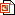Excel tutorial for creating trend lines and calculating rates of changeExcel tutorial for creating trend lines and calculating rates of change
Powerpoint of an excel tutorial for creating trend lines and calculating rates of change

File 228287 is a 1.9MB PowerPoint 2007 (.pptx)